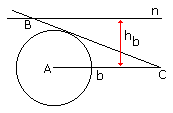The many ways to construct a triangle III

• $A, h_{b}, h_{c}$

A right triangle is well defined by a leg and an angle. Therefore, starting with an angle $A,$ we may first construct triangle $AH_{c}C$ to obtain vertex $C,$ and, in a similar manner, the triangle $AH_{b}B$ to obtain vertex $B.$

• $A, a, h_{a}$

Construct an arc (part of a circle) subtending segment $BC = a$ such that for every point $A$ on it, angle $BAC$ equals the given angle $A.$ Draw a line parallel to $BC$ at the distance $h_{a}.$ There could be $0,$ $1,$ or $2$ points of intersection of the line with the arc. In the latter case solutions are mirror images of each other; so even then there is at most one solution.

• $h_{a}, h_{b}, h_{c}$

Since $S = 2ah_{a} = 2bh_{b} = 2ch_{c},$ $a:b:c = 1/h_{a} = 1/h_{b} = 1/h_{c}.$ Since the heights are given, we may construct a triangle similar to the sought one. Consider in thus constructed triangle one altitude, say, $h_{a'}$ and apply similarity transformation with the ratio $h_{a}/h_{a'}.$• $h_{a}, h_{b}, b$

Let $AC$ has length $b.$ Draw a line $n$ parallel to $AC$ at the distance of $h_{b}.$ Draw a circle with center at $A$ and radius $h_{a}.$ Let $B$ be the intersection if line n with the tangent to the circle drawn from $C.$

• $R, a, b$

Draw a circle of radius $R$ and, in it, two chords that share an end.

• $h_{a}, h_{b}, m_{a}$We may construct $\Delta AM_{a}H_{a}.$ Since $ah_{a} = bh_{b},$ we know the ratio $a/b.$ Therefore, we may also think of the ratio $AB/M_{a}B$ as given (it's just half of $a/b.)$ Now, focus on the median $AM_{a},$ or rather on its two endpoints. The two distances from the point $B$ two $A$ and $M_{a}$ are at a given ratio. Now, this is a known fact (which deserves to be considered in its own right) that the locus of point $B$ the distance from which to the two given points is at a fixed ratio is a circle (the circle of Apollonius, unless, of course, the aforementioned ratio is $1,$ in which case the locus is a straight line.) The center of the circle lies on the line connecting the two given points and its center and radius are easily found by seeking its diameter on that line.

Point $B$ is then found at the intersection of the circle with line $M_{a}H_{a}.$

• $a, b, l_{c}$With the center $C$ draw three circles of radii $a,$ $b,$ and $l_{c}.$ For the simplicity sake, the circles will be denoted by their radii: circle $a,$ circle $b,$ and circle $l_{c}.$ We are looking for a segment $AB$ (with $A$ on circle $b$ and $B$ on circle $a)$ that is cut by circle $l_{c}$ in the ratio $a/b$ (recollect one of the basic properties of angle bisectors.)

Let $B$ be an arbitrary point on circle $a.$ Perform homothety (central similarity transformation) with center $B$ and the coefficient of similarity $a/(a+b)$ on circle $b.$ We'll get the blue circle with radius $a'.$ The blue circle intersects circle $l_{c}$ at point $L_{c}$ (and, generally, at a second point.) Draw the line $BL_{c}$ and continue it beyond $L_{c}$ to the intersection with the circle $b$ at point $A.$

Note that, since $L_{c}$ is collinear with $A$ and $B$ and lies on the blue circle, point $L_{c}$ is the image of point $A$ under the homothety. It follows that $BL_{c}/BA = a/(a+b).$ But then $L_{c}A/BA = b/(a+b),$ so that $BL_{c}/L_{c}A = a/b.$ Having this property of $L_{c}$ in mind we can prove that $\Delta ABC$ solves our problem.

Indeed, its two sides $AC$ and $BC$ have the given lengths $b$ and $a,$ respectively. Segment $CL_{c}$ has the length $l_{c}$, and the only thing we have to show is that $CL_{c}$ is the bisector of angle $C.$ We know that, for the bisector, the point $L_{c}$ divides the side $AB$ in the ratio $a/b.$ Since, on $AB,$ there is only one such point, the point defines the bisector uniquely. But, as we have seen, our point $L_{c}$ has exactly that property. Therefore, by construction, the line $CL_{c}$ is indeed the bisector of angle $C.$

France Dacar has supplied an alternative construction and rightly observed that the diagram above is not to scale.

• $A, B, l_{c}$

Two angles uniquely define a family of similar triangles. Construct any member of the family. Draw the angle bisector $l_{c}.$ If it's of the correct length, we are finished. Otherwise, the ratio of this bisector to the given one defines the coefficient of proportionality to apply to our triangle in order to get a similar one in which the bisector $l_{c}$ is of right length.

• $A, h_{a}, p$Assume we managed to construct $\Delta ABC.$ Extend $BC$ to $DE$ adding $BD = AB$ and $CE = AC.$ Thus $DE = p.$ Both $\Delta ABD$ and $\Delta ACE$ are isosceles. From here, $\angle EAC = \angle ACB/2$ and $\angle DAB = \angle ABC/2.$ Summing up, $\angle DAE = A/2 + 90^{\circ}.$

With a reference to another construction, construct $\Delta ADE.$ On $DE,$ find points $B$ and $C$ such that $\Delta ABD$ and $\Delta ACE$ are isosceles. (Draw perpendicular bisectors of $AD$ and $AE$ till they intersect $DE.)$ $\Delta ABC$ solves the problem.

• $A, O, H$

(By jack202.) If we know the position of $A,$ $O,$ $H$ in a triangle we can trace

1. The circumcircle $D$ (center $O)$ and radius $OA$

2. The 9-point circle $E$ (the center is the midpoint of $OH,$ the circle passes trough the midpoint of $AH,$ or has the radius which half that of $D.)$

The line through $A$ and $H$ intersects $E$ in a point $(F)$ that is the feet of the altitude $AH.$ Now trace the perpendicular $r$ to $AH$ trough $F.$ It will intersect $D$ at the vertices $B$ and $C.$